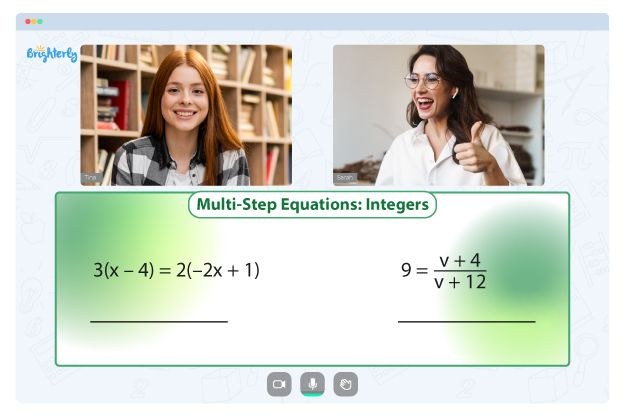# Adding And Subtracting Integers Worksheets

As kids advance in grade classes, they are expected to understand concepts like integers. This math skill is crucial within and outside the classroom; hence, tutors and parents ensure that students master them. The most straightforward way of learning the concept is using interactive adding and subtracting integers worksheets.

## Free add and subtract integers worksheet

Brighterly has several integer worksheets, all having the same purpose – helping kids master integers. Like other worksheets, adding and subtracting integers worksheets are designed by top educators with years of tutoring kids. Hence, worksheets are structured to boost students’ understanding of the topic.

Adding and subtracting integers are significant mathematical concepts. Therefore, practicing to add and subtract integers will strengthen the ability of kids to solve several mathematical problems that are related to it.

Adding and subtracting integer worksheets let kids approach questions in a stepwise manner, focusing on the easier ones first. These math worksheets are also supported by visuals, making the learning experience fun and engaging.

The adding and subtracting integers worksheet PDF resources are interactive; they consist of numerous pictures of everyday objects and colorful graphics kids can relate to. Colorful graphics are essential to capture the attention of kids. Youngsters can also use an adding and subtracting integers worksheet with answers pdf to check if they correctly solve questions.

The questions in the adding and subtracting integers worksheets are structured from the easiest to the most complicated ones. That way, kids start by learning the fundamentals and will be eager to learn more. Also, the worksheets are suitable for kids of all grades and grasping capabilities.

Math for Kids

Is Your Child Struggling With Math?
1:1 Online Math Tutoring## Benefits of integer addition and subtraction worksheet

There are several benefits of using фт adding and subtracting integers worksheet. Below are some of them:

### Development of pupils’ foundational math abilities

Using worksheets while solving math problems involves breaking down the question before using reasoning to arrive at a solution. This practice lays the groundwork for developing problem-solving skills crucial for success in more advanced mathematics.

### Real-time application

When kids practice adding and subtracting integer worksheets, they will learn to adopt the concept in real life. For instance, practicing and understanding integers with these worksheets will help your kids in everyday scenarios like counting, etc. When kids become older, they’ll employ their arithmetic abilities in practical situations like budgeting and meal planning.

### Development of logical reasoning and imagination

Students will develop their analytical thinking skills by working through the challenges in the addition and subtracting worksheets. Practicing with the worksheet will train their brains to take a more objective look at events and situations before arriving at a rational, reasoned response.### Adding And Subtracting Integers Worksheets PDF### Adding And Subtracting Integers Worksheets PDF

Adding And Subtracting Integers Worksheet Pdf### Adding And Subtracting Integers Worksheets PDF### Adding And Subtracting Integers Worksheets PDF

As students continue to practice adding and subtracting integers worksheets, they will gain self-confidence and problem-solving skills, which will come in handy as kids advance in learning math.

## Printable adding and subtracting integers worksheets

There are adding and subtracting integers worksheets with answers PDF on the Brighterly website. You can download these materials to help kids practice and teach them to learn independently. Downloading and printing a worksheet will save you the stress of going online anytime you wish to teach your kids.

### More Integers Worksheets

Need help with Equations?• Is your child finding it challenging to grasp equations concepts?
• An online tutor could be the answer.

Does your child struggle with understanding of equations? Try learning with an online tutor.